• +91 9971497814
• info@interviewmaterial.com

# RD Chapter 32- Statistics Ex-32.4 Interview Questions Answers

### Related Subjects

Question 1 :

Find the mean,variance and standard deviation for the following data:
(i) 2, 4, 5, 6, 8, 17

(ii) 6, 7, 10, 12,13, 4, 8, 12

(i)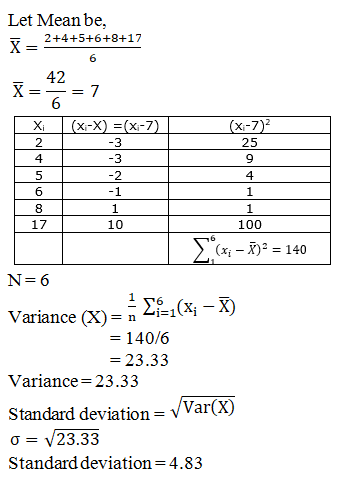(ii)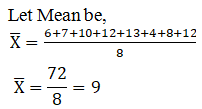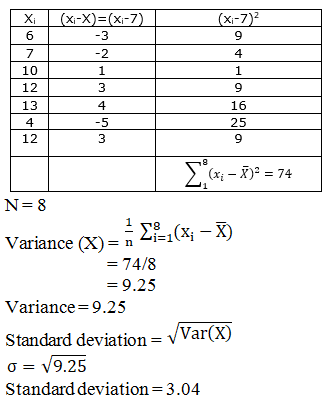Question 2 : The variance of 20 observations is 4. If each observation is multiplied by 2, find the variance of the resulting observations.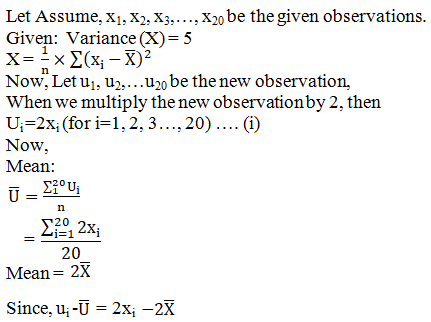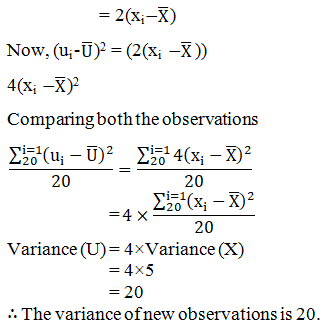Question 3 :

The variance of 15observations is 4. If each observation is increased by 9, find the variance ofthe resulting observations.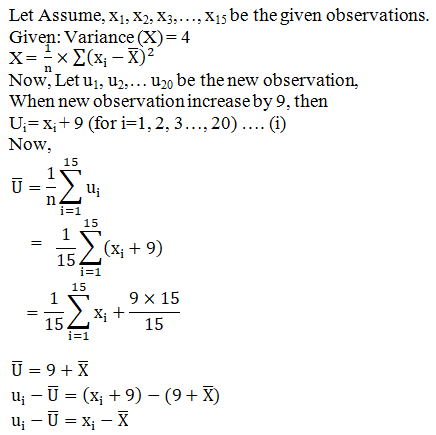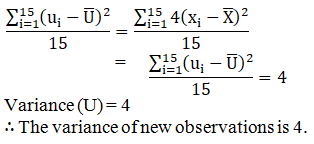Question 4 :

The mean of 5observations is 4.4 and their variance is 8.24. If three of the observationsare 1, 2 and 6, find the other two observations.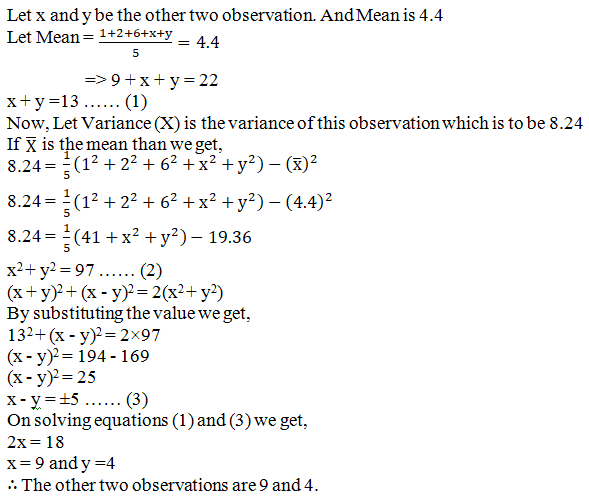Question 5 : The mean and standard deviation of 6 observations are 8 and 4 respectively. If each observation is multiplied by 3, find the new mean and new standard deviation of the resulting observations.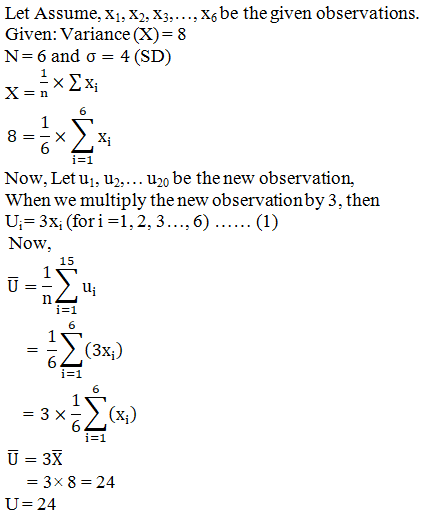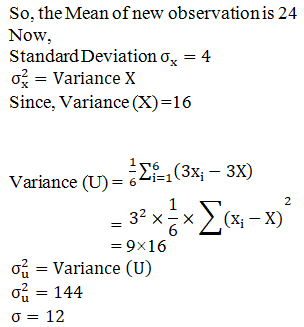Themean of new observation is 24 and Standard deviation of new observation is 12.

Question 6 : The mean and variance of 8 observations are 9 and 9.25 respectively. If six of the observations are 6, 7, 10, 12, 12 and 13, find the remaining two observations.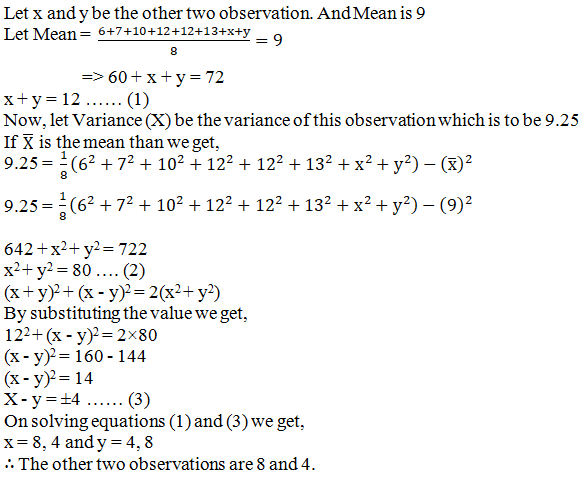Todays Deals### RD Chapter 32- Statistics Ex-32.4 Contributorskrishan

Name:
Email:

# Latest News# 9000 interview questions in different categories